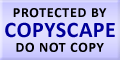Submit Assignment From Here
Name
:
Email
:
Phone
:
Country
:
Willing to pay US\$
:
Subject
:
Topic
:
Levels
:
:Hours
:

# Help with Types of Statistics

There are mainly two types of statistics, descriptive statistics and inferential statistics. Descriptive statistics are numerical values whereas inferential statistics are diagrammatical representations. Descriptive statistics contains the description of data in many forms and by use of tables, graphs, and summary measures. We can understand with an example. The population of USA in 1965 was 180,232,000; in 1975 it reached 210,203,000; in 1985 it was 238,456,000; in 1995 it was 265,987,000; and 300,101,000 in the year 2005. This information is descriptive statistics. It is descriptive statistic if we evaluate the growth of each decade in percentage. On the other hand if we use the above statistics to estimate for just one decade only. This is because in the above case we are not using past summaries but only one decade’s data.
Inferential statistics is a method by which population is described on the basis of sample result. This is also called statistical inference. It is finding a variable from the population by way of sampling method. For example a survey shows 54% of students can solve mathematics problems which include fractions, and percentages. And out of the above given percent age only 46% can solve the above problems correctly.

 Click here to submit assignment Statistics Homework Help Confidence Interval Estimation Continuous Probability Distribution Methods of Least Squares and Curve Fitting Estimation Theory Numerical Measures Principles of Data Reduction Properties of Random Sample

### Live Online Tutoring### Make Payment OnlineUS